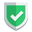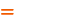我们相信：世界是美好的，你是我也是。平行空间的世界里面，不同版本的生活也在继续...

## 不同维度不同结果

``````import numpy as np
a1 = np.array( [1, 2, 3] )
a2 = np.array( [[1, 2, 3]] )
a3 = np.array( [[[ 1, 2, 3 ]]] ) ``````
``````print( a1.ndim )        # 1
print( a1.T )``````
``````1
[1 2 3]``````
``````print( a2.ndim )        # 2
print( a2.T )``````
``````2
[

]``````
``````print( a3.ndim )        # 3
print( a3.T )``````
``````3
[[]

[]

[]]``````

## 一维`ndarray`数组翻转

``````import numpy as np
a1 = np.array( [1, 2, 3] )
print( a1 )
print( a1.T )``````

``````[1 2 3]
[1 2 3]``````

## 二维`ndarray`数组翻转【常见】

``````import numpy as np
a2 = np.array( [[1, 2, 3],[4, 5, 6]] )
print( a2 )
print( a2.T )``````

``````[[1 2 3]
[4 5 6]]

[[1 4]
[2 5]
[3 6]]``````

## 三维`ndarray`数组翻转【本文重点】

``````import numpy as np
a3 = np.arange(27).reshape(3,3,3)
print( a3 )
print( a3.T )``````

``````[[[ 0  1  2]
[ 3  4  5]
[ 6  7  8]]

[[ 9 10 11]
[12 13 14]
[15 16 17]]

[[18 19 20]
[21 22 23]
[24 25 26]]]``````

step1: 第一层保持1轴不变，2轴位置换成0轴，也就是说：以顺着1轴箭头的方向看的话，2轴逆时针旋转到0轴的位置。也就是说第一层的所有数字由水平变成竖直。（原有层保持不变，数字与层脱钩）
step2：第二层重复第一层的动作，顺着1轴箭头看的话，第二层在第一层的左侧。同时，两层被竖起来的层底部被对齐。
step3：第三层重复第二层的动作。
step4：废弃被旋转的0轴，1轴，2轴概念，使用原来的坐标体系及层体系。读取数字，得到矩阵。

``````[[[ 0  9 18]
[ 3 12 21]
[ 6 15 24]]

[[ 1 10 19]
[ 4 13 22]
[ 7 16 25]]

[[ 2 11 20]
[ 5 14 23]
[ 8 17 26]]]``````

## 特别提示

• 如果理解为二维数组，那么就是0轴行，1轴列。
• 如果理解为三维矩阵，那么就是1轴行，2轴列。0轴给了新多出来的竖直轴。

## 结束语如果本文对您有帮助，或者节约了您的时间，欢迎打赏瓶饮料，建立下友谊关系。本博客不欢迎：各种镜像采集行为。请尊重原创文章内容，转载请保留作者链接。【福利】 腾讯云最新爆款活动！1核2G云服务器首年50元！【源码】本文代码片段及相关软件，请点此获取更多信息【绝密】秘籍文章入口，仅传授于有缘之人python